Experimental Tests
Invalidating
Einstein's Relativity
Go to: Next series of questions
Previous series of questions
Series #2 -      Tests Invalidating  Relativity
-----------------------
Question - (2-A)
Why should we believe that Einstein's theory of relativity is wrong?
A. - Because several experiments have shown very clearly that the results are not compatible with Einstein's predictions of general relativity.  One can also think of other important reasons, (like the non-conservation of momentum and mass-energy in general relativity [Ref.]) but this has been discussed elsewhere (see Einstein's Theory of Relativity versus Classical Mechanics).
-----------------------
Question - (2-B)
Can you give an example of an experiment giving results which are contrary to Einstein's predictions?
A. - Yes. One of the most simple and remarkable experiment showing that Nature is not compatible with Einstein's hypothesis is the one described by Sagnac in 1914. (Ref. Sagnac M. G., J. de Phys., 1914, 4, 177-195).
-----------------------
Question - (2-C)
Can you give a simple description of the Sagnac's Experiment?
A. - Yes. Here is a simple description.
Let us assume that you have a source of light (a laser) at a certain location on Earth (e.g. your home). You project that beam of light directly toward a satellite in orbit around the Earth on which there is a reflecting mirror. After that first reflection, light reaches other mirrors in space so that it can finally complete a full turn around the Earth and reach again the same spot on Earth where light has been emitted.
Using that apparatus, you can measure the time it takes for light to make a complete turn around the Earth when light moves from West to East. Now, if you repeat the same experiment, with the same mirrors, (or even do it simultaneously), using light moving from East to West, you find that the time taken by light to move from East to West is shorter than the time taken for light to complete the revolution in the opposite direction. That difference of time is observed with the same apparatus, making simultaneous measurements, at the same location. One can show that this difference is due to the Classical Newtonian motion of Earth rotation. That difference of time for light taken between each direction depends directly on the velocity of the Earth moving around itself.
Einstein's Theory claims that there is no possible way to detect the ABSOLUTE velocity of the Earth. The very use of the expression RELATIVITY comes from Einstein's hypothesis that parameters, like VELOCITY, are relative so that any absolute motion (like absolute velocity) is meaningless. However, we see above, that the velocity of Earth is responsible for the change of time light takes to go around the Earth. From a fixed location on Earth, we can detect the Earth's motion. Therefore, contrary to Einstein principle of relativity, the velocity of light is not relative to the observer. One must conclude that the Sagnac effect contradicts Einstein's hypothesis of General Relativity.
Let us note that in practice, the Sagnac effect is observed quite easily. In order to observe that effect, you do not have to make a full circle around the Earth. If you make light rotate around a diameter of only one meter and many rotations, (in either directions) you will observe the same effect. The slight change of time interval to travel in either directions can be detected using interference patterns between the beams moving simultaneously in opposite directions. This method is very sensitive to detect a very small rate of rotation. Such an observation is very important in ships (or spaceships) and in airplanes. It is a kind of optical gyroscope (laser gyroscope) manufactured commercially in large number. This optical gyroscope detects the absolute velocity of the rotating Earth from any location on Earth, even if Einstein's theory claims that light travels with respect to the observer.
There is also a Straight-Line-Component Sagnac effect. (see Einstein's Theory of Relativity versus Classical Mechanics, chapter 9).  This will not be developed here. The Sagnac effect has been discussed in many papers. Recently, A. Kelly (The Sagnac Effect and GPS Synchronization of Clock-Stations) presented a paper at the meeting of "Galileo Back in Italy", Bologna May 1999. Ref. A. G. Kelly, HDS Energy Limited Celbridge, Co, Kildare, Ireland. The Sagnac effect on the GPS Clock synchronization is extremely important.
One can conclude that you can possess now your own instrument that measures the ABSOLUTE velocity of rotation of the Earth, which is in striking contradiction with Einstein's Theory of Relativity, while most of the scientists still refuse to accept that this can actually work. This is the most striking proof that Einstein's Theory of General Relativity is wrong.
-----------------------
Question - (2-D)
Since the Sagnac experiment was known in 1914 (even before the development of general relativity), how can Einstein's general relativity be accepted so readily around 1919?
A. - For the same reason that in Galileo's time, when people refused to accept that it was not the Sun (nor the sky) which is moving around the Earth.
During Galileo's time, the interpretation of the bible was that EVERYTHING in the sky was moving around the Earth. However, a contrary observation was already definitely observed using Galileo's first telescope (when observing the satellites of Jupiter moving around Jupiter). It is reported that the monks even refused to look through the Galileo's telescope. Modern physics is dogmatic and possesses many characteristics of a religion.
-----------------------
Question - (2-E)
How accurate are the measurements of the Sagnac effect?
A. - The Sagnac experiment has been measured a large number of times during the last century. In the case of an experiment of radio waves moving around the Earth, Michelson and Gale (Ref. Michelson A. and Gale H. Astroph. J. 1925, 61, 137-145) made the first observation that the electromagnetic signals around the world took a different time in the East and West directions in 1925. Very recently, using a ring laser, Bilger H. R. et al. (IEEE Trans. 44, No:2, p. 468-470) confirmed that the Sagnac effect to better than one part in 1020.
-----------------------
Question - (2-F)
Since the measured velocity of light is different in the Sagnac experiment, what is that velocity of light?
A. - Let us consider the Earth equator as a rotating disk. Let "R" the radius of the disk and "w " the angular velocity of rotation of the disk (Earth equator). The velocity V of the observer (with his clock and his measuring meter) at distance R from the center is:1
Elements of the Problem.
We have seen in the book: "Einstein's Theory of Relativity versus Classical Mechanics that due to the velocity of the observer's frame, and as a consequence of the principle of mass-energy conservation, the unit of length changes (see equation 3.23) in the same proportion as the local clock rate (equation 3.10). Consequently, the velocity (which is the quotient of these two numbers) measured by the moving observer is represented by exactly the same number of local meters per local second. The measured velocity is the same whether we use rest units Vrest or moving units Vmov. This gives: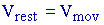2
We note also a centrifugal force at the location where the experiment is done. However, since the radius R of the disk is considered constant, and a "force" alone is not "energy", the radial acceleration (gravitational or centrifugal) without displacement is irrelevant. There is no change of energy due to the centrifugal or gravitational force alone. Any change of energy (which is the only thing relevant here) is due to the increase of tangential velocity.
Due to the velocity of the rotating disk, the matter at the outer part of the disk should be dilated as calculated by equation 3.23 This means that the local Bohr radius (of atoms) is larger, due to the kinetic energy. However, since the radius of the disk R is constant, the geometrical perimeter 2pR is also constant. Consequently, the natural expansion of matter due to the increase of Bohr radius will produce a force of compression on the atoms of the disk at the radius R. This phenomenon is similar to what happens when we squeeze matter. The inter atomic distance is reduced due to the pressure, as calculated in classical mechanics with the Young modulus. Of course, "space contraction", claimed in many papers, does not make sense.
Measured Velocity of Light for the Moving Observer on a Rotating Disk.
Let us now calculate the time taken by light to complete a full circle around the rotating disk.   When the disk is stationary, light makes a full rotation around the disk after a time interval "t" equals to: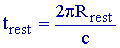3
Where c is the velocity of light when the observer is at rest, and Rrest is the radius measured at rest.
Let us calculate the time interval when both light and the observer's velocity make an anti clockwise rotation. Since "light" and the "observer" travel in the same direction, an observer at rest finds that "light" must travel a little more than a full circumference in order to reach again the observer who moved while light completes the circle.  That observer (at rest) observes that the relative velocity between "light" and the moving observer is (c-V). Since the circumference is 2pR, the time interval "trest" taken by light between two passages in the same neighborhood of the moving observer is: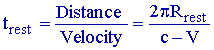4
The moving observer is also making the same measurement. He uses the moving observer's local clock giving tmov and the moving standard meter units to measure Rmov.
We have seen above (and also in the book) that between frames, the clock rate changes in the same proportion as the units of length. Furthermore we have seen (equation 2 above), that all measurements of the velocity are the same in any frame. Consequently, equation 4 becomes:5
Let us calculate the "change" of time interval (tmov) as a function of the velocity of the rotating disk. When the velocity V of the rotating disk changes from zero to V, the difference of time interval is given by the derivative, which is:6
Since all units used below will always be from the moving frame, we will now simplify the notation without writing the sub index (mov). The disk (Earth equator) has an area "A" equal to:7
From equation 1, we have: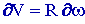8
Taking into account that the velocity V is very small with respect to the velocity of light, we see in equation 6 that V2 and cV is negligible with respect to c2.  Using t equals Dt, for a change of angular velocity w (with respect to an initial value w=0), equations 7 and 8 in 6 give:9
Equation 9 gives the difference of time interval Dt, taken by light to complete a full rotation around the disk due to the change "¶w " of angular velocity from zero to w.
Equation 9 is identical to Sagnac equation (Sagnac M. G. J. de Physics., 1914, 4, 177-195). Sagnac equation gives a double amount as expected, because it represents by definition, the difference of time interval between two beams traveling simultaneously in opposite directions, corresponding to a change of angular velocity from -w to +w . It has been clearly measured and demonstrated (see question 2-E above), that the Sagnac equation is in perfect agreement with observations.  More discussion is given below.
Velocity of Light.
In order to determine the "measured " velocity of light (called "v") as found experimentally by the moving observer, we compare the time taken by light to complete a rotation around the disk (at velocity c) with the difference of time interval given by equation 9. The circumference is: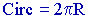10
The time To taken to complete a rotation when the disk does not rotate is:11
The time Tv taken when the disk rotates at velocity V (in the same direction as light) using equation 9 is:12
Equation 11 in 12 gives:13
The measured velocity of light "v" as seen by the moving observer is then: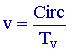14
Equations 10 and 13 in 14 give:15
where16
Since e <<1, equation 15 gives: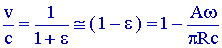17
Using equations 1, 7 in 17, we find: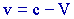18
Equation 18, shows that the measured velocity of light "v" for an observer moving at velocity "V" is equal to the difference (or the sum) of the velocities "c-V" (taking into account the direction of V).
This is not only in perfect agreement with the Sagnac experiments, but also agrees with the results expected from an absolute frame of reference, in which light is traveling at velocity c with respect to a fixed frame at rest.  Equation 18 shows that for a circular trajectory, the measured velocity of light is not "c" but "v", in the frame of reference used by the observer moving with the disk. The velocity of light v is equal to (c-V) or (c+V) depending on the relative direction of V with respect to c, as expected "logically" in classical mechanics. This is the result obtained when light is not reflected back toward the initial direction.
Compatibility with the Measurement of a Reflected Beam of Light.
When the velocity of light is measured after being reflected back on a single line toward the original location, (as measured most of the time), we have seen  that the velocity of light appears constant (equal to c) to any moving observer. It is erroneously believed(see chapter nine) that this result is not compatible with classical mechanics in which the predicted velocity of a wave depends on the relative velocity of the observer with respect to the medium (according to the relationship c-V or c+V).
As demonstrated previously, the measured velocity of light between frame is also compatible with the existence of an absolute frame of reference with an absolute velocity of light c in a fixed frame.  This is because the clock rate and the standard meter are naturally but automatically modified (due to mass-energy conservation) to compensate for the velocity of the frame. This agreement is fully understood after considering the clock synchronization explained in chapter 9 of the book: Einstein's Theory of Relativity versus Classical Mechanics.
Absolute frame of reference.
Whatever the method of measurement is, we have seen that all results are compatible with a constant velocity of light moving in an absolute frame of reference. The measured velocity of light is compatible with a fixed frame of reference, either using the circular trajectory of light as done using the Sagnac experiment or using the velocity of light after reflection on a mirror.
It is clear that the physics of the 20th century has not been able to realize that, due to mass-energy conservation, the measuring rods and the clock rate of the linearly moving observers change in such a way that the velocity of light becomes independent of the proper velocity V of a linearly moving observer.  However, this natural compensation does not exist in the case of a circular motion of light as shown above, but the result is still compatible with the same constant velocity with respect to an absolute frame.  The scientists have been brainwashed to such a degree that they even reject the argument without reading the papers just as in Galileo's time.
It is important to notice that all these results have been confirmed experimentally.  In the case of circular trajectories Sagnac (Sagnac M. G. J. de Physics., 1914, 4, 177-195) has shown how the velocity of light varies with the observer's velocity.  Sagnac equation has been verified experimentally in numerous circumstances. It is the principle involved in the optical gyroscopes. This is explained above in question 2-E.  Furthermore, Sagnac equation is equally needed in the mathematical relationships that gives the exact international time GPS (Global Positioning System) using orbiting atomic clocks in satellites. The GPS system needs the "Sagnac correction" in their computer program to calculate the exact GPS time.
This shows that the absolute velocity of light "c" with respect to a fixed frame in space is an experimental fact. The results are compatible with all known experiments.  More general information is given in the book; "Einstein's Theory of Relativity versus Classical Mechanics".
-------------------------
Question - (2-G)
Einstein proposed the hypothesis that the force of acceleration G due to gravity is identical to the force due to inertia (when the velocity of a mass is changed). According to Einstein, these two forces are indistinguishable. Are there experiments showing that this is wrong?
A. - Yes, several experiments show that those two forces have a different nature, because they lead to different results. The force of gravity can be applied during a long time without requiring energy. However, the acceleration involving a change of velocity requires energy. It is well known that they lead to quite different consequences that can be verified experimentally.
-----------------------
Question - (2-H)
Can you give an example showing that the force of acceleration due to Gravity leads to consequences which are different from the acceleration due to a real change of velocity of a mass ?
A. - Let us compare the consequences of Inertial and Gravitational Accelerations.
Consider a mass standing on the surface of the Earth. After one year, the mass submitted to gravitational acceleration is always standing there, without changing its energy or velocity. Let us also consider another identical mass, submitted to an inertial acceleration of one G in outer space due to the push of a rocket. After one year, the enormous energy given to the mass is such that its velocity has reached an important fraction of the velocity of light. If that mass falls on Earth its energy produces a gigantic crater on the impact. Einstein's theory claims that these two phenomena are equivalent and indistinguishable. This is non-sense. Any scientist should be able to see the difference. In order to convince people, Einstein adds that there is no difference for the observer located in the moving mass' frame of reference.
In order to illustrate Einstein's argument, let us consider another case in which relative motion is observed. A well known example is when we observe the Sun and the stars crossing the sky everyday, (or the sunrise or the sunset). Relative to the Earth surface, the sky is moving around us and the Sun disappears below the horizon. However, we all know that Galileo, using astronomical data, observed that in fact, it is the Earth that rotates and not the Sun (and the sky). Using external astronomical information, Galileo found the correct motion of the Earth, which led him to correct our understanding of the motion of planets in the solar system. The simple observation of the relative motion of the Sun and stars around us, which previously led to the pre-Galilean naive claim of the motion of the sky around us, was very poor science. GOOD SCIENCE TAKES INTO ACCOUNT ALL POSSIBLE OBSERVATIONS AVAILABLE. It is very bad science to ignore voluntarily some of the information available.
In the case of the inertial acceleration of the mass during one year, it is bad science to ignore the fact that the mass submitted to an inertial acceleration needs energy for that acceleration and will restore later the energy acquired. The mass accelerated by the rocket has acquired energy and an increase of "mass equivalent" (m=E/c2) mass cannot logically be ignored.
One must conclude that the Einstein's principle of equivalence between inertial and gravitational acceleration leads to a catastrophic physical incoherence between inertial systems. Einstein's equivalence principle between inertial and gravitational forces is not compatible with coherent observations. This principle of equivalence between inertial and gravitational mass is bad science belonging to pre-Galilean epoch.
<><><><><><><><><><><><>
New  Choice of Questions

<><><><><><><><><><><><><>

<><><><><><><><><><><><><>

invalidation.html           Series 2            Tests Invalidating Relativity           July, 1999